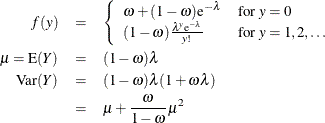# The HPGENSELECT Procedure

### Response Probability Distribution Functions

Subsections:

#### Binary Distribution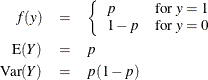#### Binomial Distribution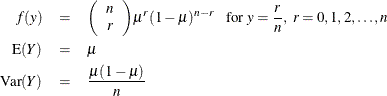#### Gamma Distribution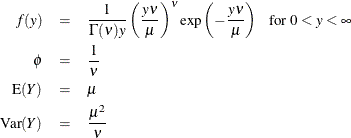For the gamma distribution,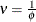is the estimated dispersion parameter that is displayed in the output. The parameter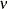is also sometimes called the gamma index parameter.

#### Inverse Gaussian Distribution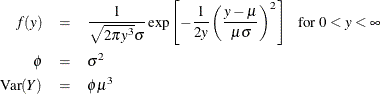#### Multinomial Distribution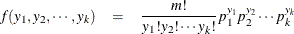#### Negative Binomial Distribution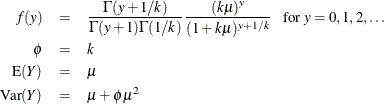For the negative binomial distribution, k is the estimated dispersion parameter that is displayed in the output.

#### Normal Distribution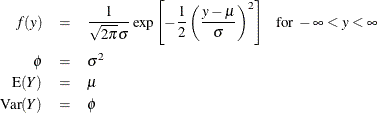#### Poisson Distribution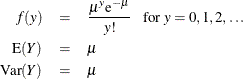#### Tweedie Distribution

The Tweedie model is a generalized linear model from the exponential family. The Tweedie distribution is characterized by three parameters: the mean parameter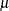, the dispersion, and the power p. The variance of the distribution is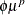. For values of p in the range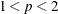, a Tweedie random variable can be represented as a Poisson sum of gamma distributed random variables. That is,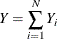where N has a Poisson distribution that has mean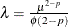and the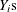have independent, identical gamma distributions , each of which has an expected value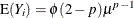and an index parameter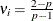.

In this case, Y has a discrete mass at 0,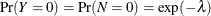, and the probability density of Y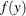is represented by an infinite series for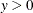. The HPGENSELECT procedure restricts the power parameter to satisfy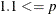for numerical stability in model fitting. The Tweedie distribution does not have a general closed form representation for all values of p. It can be characterized in terms of the distribution mean parameter, dispersion parameter, and power parameter p. For more information about the Tweedie distribution, see Frees (2010).

The distribution mean and variance are given by: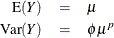#### Zero-Inflated Negative Binomial Distribution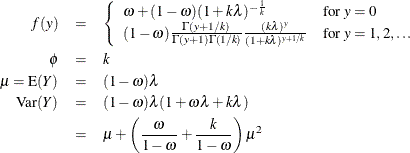For the zero-inflated negative binomial distribution, k is the estimated dispersion parameter that is displayed in the output.

#### Zero-Inflated Poisson Distribution